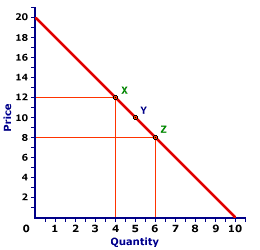Friday  December 1, 2023
 AmosWEB means Economics with a Touch of Whimsy!LOGROLLING: A systematic exchange of votes by politicians to obtain approval of specific legislation. That is, Senator Grapht agrees to vote for Senator Brybe's pet project if Senator Brybe votes for Senator Grapht's favorite piece of legislation. Such logrolling can be explicit or implicit. The explicit kind involves two separate bills, in which each politician is forced to "go on record" with a vote. The implicit kind, which many politicians favor, is where several separate programs are wrapped into a single bill. Every politician can then tell the folks back home that they really only wanted the "one thing" that helped their constituencies the most, but had to vote for "other things" as well. Logrolling is big reason our government is big and prone to inefficiency.ARC ELASTICITY:

The average elasticity for discrete changes in two variables. The distinguishing characteristic of arc elasticity is that percentage changes are calculated based on the average of initial and ending values of each variable, rather than initial values. Arc elasticity is generally calculated using the midpoint elasticity formula. The contrast to arc elasticity is point elasticity. For infinitesimally small changes in two variables, arc elasticity is the same as point elasticity.
Arc elasticity is best considered the average elasticity over a range of values for a relation. Like any average, some values within the range are likely to be greater and some less. However, it provides a quick approximation of elasticity when more precise and sophisticated calculation techniques are not possible.

### Working Through an Example

A Standard Demand CurveThe demand curve displayed to the right can be used to illustrate the measurement of arc elasticity using the midpoint elasticity formula. If the price declines from \$12 to \$8, the quantity demanded increases from 4 to 6, from point X to point Z. Using this midpoint formula (with price designated as P and quantity designated as Q) average price elasticity of demand is:
 midpointelasticity = (Q[Z] - Q[X])(Q[Z] + Q[X])/2 ÷ (P[Z] - P[X])(P[Z] + P[X])/2

 midpointelasticity = (6 - 4)(6 + 4)/2 ÷ (8 - 12)(8 + 12)/2 = (2)(5) ÷ (-4)(10)

 midpointelasticity = 0.4 ÷ -0.4 = -1

Ignoring the minus sign, the price elasticity of demand over this segment of the demand curve from X to Z is 1.0.

### An Average Value

This value of 1.0 is actually an average for the entire range between points X and Z. Precise estimates of point elasticity shows that the elasticity is 0.67 at point X and 1.5 at point Z. Moreover, the elasticity is different at each point on a straight line demand curve such as this one. The only point in which the elasticity is exactly equal to 1.0 is at point Y, the midpoint between X and Z.

This last observation is worth emphasizing. The midpoint elasticity formula effectively estimates the point elasticity at the very midpoint of the overall segment. This means that the elasticity of any point on a demand curve (point elasticity) can be obtained by calculating the arc elasticity with the midpoint elasticity formula such that the desired point is dead center in the middle, the midpoint of the arc.

 <= AMERICAN ECONOMIC ASSOCIATION ASSUMPTION =>Recommended Citation:

ARC ELASTICITY, AmosWEB Encyclonomic WEB*pedia, http://www.AmosWEB.com, AmosWEB LLC, 2000-2023. [Accessed: December 1, 2023].

Check Out These Related Terms...

Or For A Little Background...

And For Further Study...
Search Again?YELLOW CHIPPEROON[What's This?] Today, you are likely to spend a great deal of time at a flea market wanting to buy either a graduation present for your niece or nephew or a toaster oven that has convection cooking. Be on the lookout for jovial bank tellers.Your Complete ScopeThe penny is the only coin minted by the U.S. government in which the "face" on the head looks to the right. All others face left."Sometimes our light goes out, but is blown into flame by another human being. Each of us owes deepest thanks to those who have rekindled this light. "-- Albert Schweitzer, missionary physicianHSBHigh School and BeyondA PEDestrian's Guide Xtra CreditTell us what you think about AmosWEB. Like what you see? Have suggestions for improvements? Let us know. Click the User Feedback link.| | | | | | | | | | |
| | | |

Thanks for visiting AmosWEB